## research papersJOURNAL OFAPPLIEDCRYSTALLOGRAPHY
ISSN: 1600-5767
Volume 39| Part 6| December 2006| Pages 842-849

## Structure matching: measures of similarity and pseudosymmetry

aUniversity of Oxford, UK, and bOxford Diffraction Ltd, UK
*Correspondence e-mail: anna.collins@chem.ox.ac.uk

(Received 14 February 2006; accepted 20 September 2006)

A sizeable proportion of structures with Z′ = 2 are thought to exhibit pseudosymmetry, but establishing the extent of the deviation from true symmetry is problematic. By considering both the conformational similarity between the independent molecules and the way in which they are related in space, assessment of the pseudosymmetry of a structure becomes possible. A method of matching two groups of atoms where both these factors are quantified using CRYSTALS [Betteridge, Carruthers, Cooper, Prout & Watkin (2003). J. Appl. Cryst. 36, 1487] is described.

### 1. Introduction

Estimates of the proportion of Z′ > 1 structures that possess pseudosymmetry elements vary between 10% (Desiraju et al., 1991) and 27% (Steed, 2003). The large difference between these values may be attributed to several factors, key amongst them being the precise definition of pseudosymmetry employed, and difficulties in identifying and quantifying pseudosymmetry for a large number of structures. Establishing and defining the extent of the deviation from true symmetry is problematic. The main difficulty lies in categorizing the structures where the conformations are the same or highly similar into those which are related by a true crystallographic operator which would require a higher symmetry space group, and those where the symmetry relationship between the two molecules is arbitrary with respect to the existing space-group operators.

Conformational similarity of crystallographically independent molecules has been the subject of several studies (see, for example, Sona & Gautham, 1992; Gautham, 1992) with the root-mean-square deviation of atomic coordinates used as a measure of similarity. This is a very useful comparison, but it does not allow the relationship between molecules, and thus the extent of pseudosymmetry, to be established.

### 2. Methodology

The task of automating the comparison of two independent groups of atoms falls into four stages.

#### 2.1. Pair-wise association of atoms

Programs like CRYSTALS (Betteridge et al., 2003) and XP (Sheldrick, 1991) contain utilities for computing a best fit' between molecules, but require the user to specify which pairs of atoms from each molecule are to be associated with each other. Programs like PLATON (Spek, 2003) and MISSYM (Le Page, 1988) do not require the user to make the pair-wise associations, but assume that the molecules being compared are sufficiently similar that a valid symmetry operator can be identified by permutation of all pair-wise associations. The first of these strategies requires too much manual intervention if more than a few structures are to be examined, and the second fails if the pseudosymmetry is only local.

#### 2.2. Computing a best fit'

Generally best' is interpreted in the least-squares sense, but even here there are a number of strategies available for mapping one set of atomic coordinates onto the other. All start by translating both molecules so that their centres of gravity lie at the origin (Diamond, 1988).

##### 2.2.1. Strategy 1: computation of a pure rotation matrix

This method could be appropriate if the material were enantiopure, so that both molecules were of the same chirality. Because the matrix is simply a rotation, the molecular geometry does not change (Kabsch, 1978). Experience suggests that for materials with few chiral centres and some torsional flexibility, the relationship between the two independent molecules is often best represented by an approximate improper operation (centre, mirror or glide). The chiral centres cannot obey the pseudo operation, but this has little influence on the overall molecular envelope [e.g. Fig. 1; Cambridge Structural Database (CSD; Allen, 2002) refcode FUGSIJ; Stensland et al., 1987]. The best proper operator is a twofold rotation, giving very poor positional matching.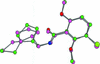Figure 1 FUGSIJ. The material is chiral in space group P21, but the achiral moieties (on the right of the picture) are related by a good pseudo mirror (x, −y, z +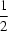).
##### 2.2.2. Strategy 2: computation of a rotation/inversion matrix

This method also preserves molecular dimensions, but permits a change of chirality, making it suitable for the comparison of racemates (Diamond, 1976).

##### 2.2.3. Strategy 3: computation of a generalized rotation–dilation matrix

This method allows an anisotropic dilation or contraction of one of the molecules. As this is quantified, it also provides a measure of the similarity of the molecules (Diamond, 1976).

#### 2.3. Assessing the best-fit' matrix

Having determined the translations and rotations/inversions which relate the two molecules, the matrix can be assessed to see whether it approximates to an operator compatible with the metric symmetry of the crystal, and is thus an approximate operator for a space group of higher symmetry. The alternative is that the operator is local and cannot be propagated.

#### 2.4. Assessing molecular similarity

Once the best fit between the molecules has been found, their conformational similarity can be assessed.

### 3. Implementation

A two-stage method has proved to be most robust.

Stage one considers the molecular structures of the groups based purely on atomic connectivity. An initial check ensures that the two molecules contain the same numbers of atoms; matching is abandoned if this is not the case. Because of the way in which H atoms are located in structure determinations, the user may prefer to use a structure exactly as published, to eliminate all the H atoms or to insert H atoms at theoretical positions. At this stage it is also possible to allow the pairing of atoms of different element types; this may be preferable under some circumstances, e.g. in comparing bromo- and chloro-analogues of a material; however, it may reduce the chance of obtaining an accurate match. A two-dimensional bonding network is computed based on standard covalent radii. The bonding network of the two groups is used to attempt to assign a unique identifier to each atom in the group. It should then be a simple step to pair up atoms from each group with matching unique identifiers.

Stage two consists of three-dimensional fitting of the atom pairs identified in stage one. If stage one yields two alternative solutions, both are tried.

#### 3.1. Assigning unique atom identifiers

Every atom in the structure is initially assigned an identifier based on its atomic number and the number of bonds it makes to other atoms (which will depend upon whether H atoms are included or not),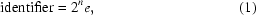where e is the atomic number (electron count) and n is the number of bonds.

Consider the structure HACTPH10/11, where the H atoms have been removed (see Fig. 2).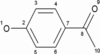Figure 2 Two-dimensional connectivity of HACTPH10/11.

The aim is to assign a unique identifier to each atom of the molecule (Table 1).

 Table 1Initial values of the identifiers for each non-H atom in HACTPH10/11
 O1 21 × 8 16 C2 23 × 6 48 C3 22 × 6 24 C4 22 × 6 24 C5 22 × 6 24 C6 22 × 6 24 C7 23 × 6 48 C8 23 × 6 48 O9 21 × 8 16 C10 21 × 6 12

In this case, several atoms have been assigned the same identifier, but by visual inspection are clearly different, e.g. C7 and C8. When this happens, the identifiers of adjacent bonded atoms are added to the identifiers of the clashing atoms. This process is looped through until either all the atoms have been assigned a unique identifier, or ten cycles have been completed with no change in the number of unique identifiers. Once an atom has been assigned a unique identifier, the identifier is not changed again. To prevent the values of the identifier overflowing the internal representation of the number within the computer, if the value of any identifier in the molecule becomes greater than 999999, all identifiers with a value greater than 9999 are divided by 10, and the process continues as before. Note that it is the unique values that are important, not their relative magnitude. For the above example, see Table 2.

 Table 2Identifiers for HACTPH10/11
Initial value First cycle Second cycle
O1 16 64 176
C2 48 112 112
C3 24 96 304
C4 24 96 336
C5 24 96 304
C6 24 96 336
C7 48 144 144
C8 48 124 124
O9 16 64 188
C10 12 60 60

After two cycles, all atoms have been assigned unique identifiers except C3 and C5, and C4 and C6. Further cycles will not resolve the situation. There is local internal structural symmetry (IS) in the bonding topology, and these atoms cannot be differentiated during the two-dimensional pairing.

In an extreme case, the whole molecule may contain internal symmetry. In such cases, there is no unique set of identifiers.

#### 3.2. Example

FIJRUL (Try et al., 1998) is a structure with twofold internal symmetry selected from the CSD (Fig. 3).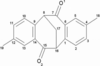Figure 3 Two-dimensional connectivity of FIJRUL, which has twofold internal symmetry.

In two dimensions the molecule possesses twofold rotational symmetry. This means that there are two possible matches: O1→O1′, O2→O2′, C1→C1′, C2→C2′ etc., and O1→O2′, O2→O1′, C1→C9′, C2→C10′ etc., where the prime denotes the second molecule. Only one atom (C17) possesses a unique identifier; all other atoms inevitably share their identifier with one other atom.

To perform an initial match, three nonlinear atoms with unique identifiers are required. If this step fails, a fragment is assigned twofold internal symmetry, if at least one atom shares its identifier with only one other atom in the group. The test continues for threefold and fourfold symmetry, after which a fragment's internal symmetry is simply assigned as lots'. The assignment of internal symmetry is not a careful assessment of a fundamental property of the bonding, but is merely a tool to decide whether to attempt three-dimensional matching at this stage.

In cases where the internal symmetry is twofold, both possible matches are passed on to the three-dimensional matching stage. When the topological symmetry is higher than two, the order of the internal symmetry is noted, but no match is performed. This is also the case if resolving the initial apparent twofold internal symmetry results in a structure which still contains internal twofold (or higher) symmetry.

#### 3.3. Fitting two groups of atoms

Once the atoms have been paired, the best fit between two sets of atomic coordinates is found. The transformation D, which rotates and translates one set of coordinates onto the other, is calculated: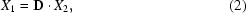where X1 and X2 are two sets of atomic coordinates (dimensions 4 × n, with the last element in each row equal to 1) and D is a 4 × 4 rotation–dilation matrix. Atoms must be in the same order in X1 and X2; hence our requirement for pair-wise matching.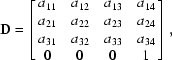where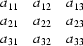is the linear transformation, and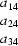is the translational component.

The computation of D can be broken down into two basic steps: calculation of the translational component and calculation of the rotational component. The calculation of the rotational component is simplified by carrying out the fit in an orthogonal coordinate system, rather than crystallographic axis system (which may not be orthogonal), as this minimizes the effects of skew and dilations of the molecular configuration. The centroids of the two molecules are computed and the molecules are moved so that both have their centroid on the cell origin.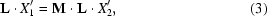where L is the orthogonalization matrix, M = LDL−1, and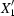and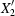are the atomic coordinates of molecules 1 and 2, respectively, when both molecules have their centroids at the origin. Note that it is arbitrary which molecule is labelled molecule 1 and which molecule 2; by default, the first atom in the atomic parameter list in CRYSTALS and all atoms in the same fragment belong to molecule 1.

M is found by post-multiplying both sides by [L]T: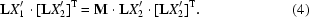Rearrangement of this equation gives: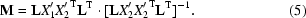The non-translational components of the matrix M can be written as a product of a pure rotation, R, and a dilation, T. The dilation can also be found from an analysis of the eigenvalues and eigenvectors of MTM (Diamond, 1976) and then R = MT−1. The pure rotation giving the best fit in the original coordinate system is given by L−1RL.

If the diagonal elements of T differ substantially from unity, then one molecule must be contracted or dilated relative to the other.

The translational component is computed from the positions of centroids of the two molecules. Once the rotational component has been computed, it is applied to the coordinates of the centroid of one molecule, and then the coordinates of the centroid of the second molecule are subtracted, i.e.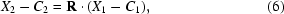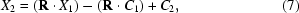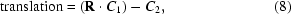where X1 and X2 are the atomic coordinates of the first and second molecules, respectively, and C1 and C2 are the coordinates of the centroids of the two molecules.

##### 3.3.1. Additional information for pair-wise association

It is not necessary for all atoms to be given unique identifiers for the match to proceed; a minimum of four atoms that are non-coplanar and have unique identifiers is required.

After the two-dimensional pairing has been completed as far as possible, the initial three-dimensional fit is carried out. The centroids and thence the principal moments of inertia of each molecule are computed. The two groups are moved so that their centroids are placed on the origin. The groups are each rotated to the best plane orthogonal system on the basis of their principal moments of inertia. The rotational component of D is computed by matching the unique atoms. The translational part of D is calculated by applying the calculated rotation to one group then subtracting the coordinates of the centroid from the coordinates of the centroid in its original position. This gives the initial fit. It may now be possible to relate atoms that could not be assigned unique identifiers at the two-dimensional matching stage. This is achieved by combining knowledge of which atoms in the two groups are closest in space with knowledge about which atoms are bonded. So in the HACTPH10 example, it may be that C3 and C3′ are closer in space than C3 and C5′, but that C4 and C6′ are closer in space than C4 and C4′. By retaining the bonding information, the most physically realistic match is obtained (Fig. 4).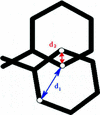Figure 4 Illustration of the importance of atom connectivity to achieve correct pairing of atoms. Here the distance d1 represents the correct pair, while the shorter distance d2 corresponds to the incorrect pair.

### 4. Similarity measures

Once the two groups have been matched, it becomes possible to identify pseudosymmetry. This is based on two criteria: the conformational similarity of two groups and the compatibility of transformation D with the space group, referred to here as the pseudo deviation. The conformational similarity is based on three values; all three values are required to be low to indicate that the molecules are pseudosymmetric.

#### 4.1. Atomic coordinate similarity

The root-mean-square deviation (RMSD) between atomic coordinates of the two molecules after fitting is the most commonly used measure of similarity between crystallographically independent molecules as it is easily derived after least-squares superposition.

The value gives an overview of how good the match between the two molecules is. A low value indicates that the match has been performed successfully; a high value indicates that the molecules are in some way different, and different measures of conformational similarity can be used to probe the nature of the differences.

When there is twofold topological symmetry in a molecule, the match with the lowest atomic coordinate RMSD is used for analysis as this gives the best indication that the match has been performed successfully.

#### 4.2. Torsional similarity

One of the major drawbacks of using simply the RMSD of atomic coordinates is that it hinders differentiating between a general small deformation over one entire molecule and the situation where differences are concentrated in a small area of the molecule, for example in the relative orientations of phenyl groups or in the conformation of a side chain. Assessment of conformational similarity is possible if the RMSDs of the torsion angles are considered. It will take high values when there are some very different torsion angles and low values when there are just small differences.

#### 4.3. Bond length similarity

For a good quality crystal structure determination, it is to be expected that the independent molecules will have equivalent bond lengths, regardless of their conformations. Thus the root-mean-square of differences in bond length represents a good check on the overall quality of the structure.

#### 4.4. Examples

##### 4.4.1. TICNOI

The structure has low bond length RMSD (0.010 Å) and torsional RMSD (1.792°), but comparatively high atomic coordinate RMSD (0.196 Å), indicating that there may have been problems in refinement. Visual inspection suggests that such problems may lie with the phenyl rings (Fig. 5).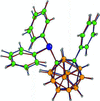Figure 5 Overlay of the matched independent molecules of TICNOI. Bonds of one molecule have been coloured red, bonds of the other molecule blue.
##### 4.4.2. CUJQIH

This is an example of a structure where there is a low torsional RMSD (1.722°), but high bond length RMSD (0.052 Å), indicating that the conformations are the same, but there is a problem with the structure overall (Fig. 6).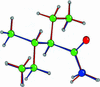Figure 6 Overlay of the matched independent molecules of CUJQIH. Bonds of one molecule have been coloured red, bonds of the other molecule blue. There is a large difference in one of the N—H bond lengths between the two molecules.
##### 4.4.3. HUSJEK

This structure has a high torsion angle RMSD (55.196°), but a low bond length RMSD (0.004 Å). This indicates that the independent molecules have different conformations, which is borne out by visual inspection of the match (Fig. 7).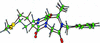Figure 7 Overlay of the matched independent molecules of HUSJEK. Bonds of one molecule have been coloured red, bonds of the other molecule blue. There is a clear difference in conformation in the carbon–sulfur chain on the left of this figure.

#### 4.5. Pseudo deviation'

In order to assess how compatible the transformation D (known as the pseudo operator) is with the operators of the existing space group and with the metric symmetry of the unit cell, the rotational components only of D are idealized' to the nearest integer, to give the closest ideal operator, OPN. By applying this operator to the existing space-group operators, a pseudo space group is generated.

Consider the calculation of the pseudo deviation for a Z′ = 2 structure in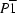. This has two general positions (M = 2), and so two symmetry operators. Applying OPN to these operators produces a further M operators. These are the operators of the pseudo space group (Table 3).

 Table 3The form of the operator array for a pseudo space group generated from a P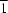cell and one additional pseudo operator, OPN
Original space-group operators Additional pseudo space group operators
Operator type Operator number Operator type Operator number
1 1 1*OPN 3 (M+1)
−1 2 −1*OPN 4 (M+2)

The matrices of the second column of the array (operators 3 and 4 for this example) are checked to see if they nearly correspond to an inversion. If an inversion is found, then for a centrosymmetric space group the pseudo operator is of a translational type (through combination with the existing inversion), while in a non-centrosymmetric space group it is of an inversion type; the latter cases are particularly interesting when the molecule is chiral.

The pseudo space group is then tested to see it if forms a closed set, i.e. when D is applied to each operator of the pseudo space group, no new operators should be generated. It is a requirement of any real space group that its operators are a closed set (International Tables for Crystallography, Vol. A, 1992). D is pre-multiplied by each of the operators of the pseudo space group in turn. The RMSD between the components of the resulting operator (RMSD-OPM) and those of each of the operators of the pseudo space group is calculated. The lowest RMSD-OPM is stored as the best match' value for that OPM, a low RMSD-OPM indicating that there is a good match between one of the operators and D. Thus a low value indicates that there is a member of the pseudo space group that is similar to D which is consistent with a closed set, while a large value indicates that D does not form a closed set in combination with the existing space-group operators. The process is then repeated for the inverse of D, D−1, as the inverse of an operator which forms part of a closed set must also be a member of that set.

Each time OPM is calculated, the value of the RMSD-OPM found is compared with the value of the best match that has been stored. If the new value is larger, then it becomes the stored value. This worst best match' value is the pseudo deviation (Δ). In most cases the rotational components of the closest ideal operator take values of 0, 1 and −1, resulting in a value of pseudo deviation that usually takes values in the range 0–1.

The periodicity of the crystal lattice is taken into consideration in the calculation of the translational components of the matrices, by putting all translations on a scale of −1/2 to +1/2. For example, translational components in two matrices of 0.01 and 0.03 in x correspond to a separation of 0.02x; this is equivalent to the separation of components of 0.01 and 0.99 in x.

#### 4.6. Example: BOMLAQ

A relationship between the two crystallographically independent molecules in the structure is found and then idealized: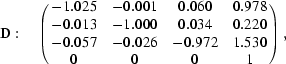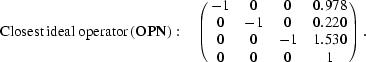The pseudo space group can now be generated. In this example the original space group is P21/n, which has a site multiplicity of four, so there will be four additional operators in the pseudo space group.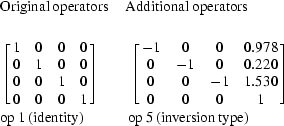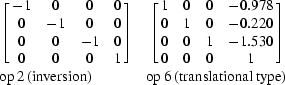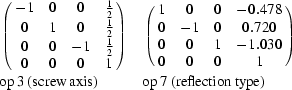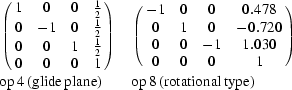The operators are now tested for a closed set, e.g.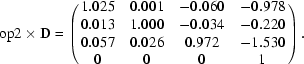The closest operator is number 6, with an RMSD of 0.1225.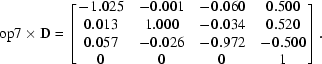The closest operator is number 3 with an RMSD-OPM of 0.0374.

Here, the highest best match' value is 0.1225, so that would be the value of the pseudo deviation.

The value of the pseudo deviation takes into account the metric symmetry of the cell. For example, in a primitive cell with a pseudo screw axis running parallel to a cell axis, the value of the pseudo deviation will become lower as the angles between that axis and the others become closer to 90°. The structure NIFPEX has a positional conformational deviation of 0.1386 Å and a pseudo deviation of 0.0192. The cell dimensions are a = 9.485, b = 10.388, c = 12.363 Å, α = 89.83, β = 106.78, γ = 90.26°. Using a CRYSTALS script, the α and γ angles were initialized to 90° and then α was increased by 0.05° increments up to 92.50°, with the match carried out for each angle. As the angle increases, so does the pseudo deviation in an approximately linear fashion.

The pseudo operator (where α = 90.00°) is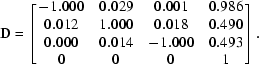The positional RMSD was 0.1352 Å and the pseudo deviation 0.0180. This corresponds to a pseudo c-glide plane at height b = 1/4, and thus the structure tends to a P21/c cell.

The final pseudo operator (where α = 92.50°) is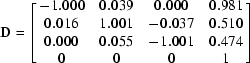The final positional conformational deviation was 0.2252 Å and the pseudo deviation was Δ = 0.0390. The results are summarized in Table 4.

 Table 4RMSD and pseudo deviation as a function of cell angle α
Cell angle, α (°) RMSD Pseudo deviation
89.8 0.1386 0.0192
90 0.1352 0.0180
92.5 0.2252 0.0390

The values of conformational deviation change because the atomic coordinates are calculated in crystal fractions, so the conformations of the two molecules will distort as the unit cell changes shape, but in slightly different ways to one another.

### 5. Example applications

#### 5.1. Pair-wise matching for atomic renumbering

A practical application of molecular structure matching is obtaining a consistent naming scheme for each molecule in the asymmetric unit. In a Z′ > 1 structure, it is useful if equivalent atoms in each formula unit have related identifiers. For the particular case of Z′ = 2, all atoms are initially identified as Q' atoms. The user then assigns systematic names of the form C1, C2 etc. to the atoms in one entity. These names can then be propagated into the second entity with the numerical part offset by some constant, e.g. C101, C102.

This operation should be performed before H atoms are automatically inserted (using the PERHYDROGENATE' command) so that the generated H atoms have identifiers related to their parent atoms.

#### 5.2. Pair-wise matching for structural comparison

Visualization of the fit between two molecules is possible by application of the transformation D to the second set of coordinates. Display in the crystal packing program Cameron (Watkin et al., 1996) reveals any important differences between the conformations (Fig. 8).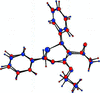Figure 8 The two independent molecules in QEQRUZ (Vigante et al., 2000) overlaid with the best match. Although the molecules are chiral in P21, the best operator is a pseudo centre.

#### 5.3. Identifying poor refinement using the structural similarity measures

It is well documented (Marsh, 1981) that a pseudo translational symmetry or a pseudo centre of symmetry can degrade a structural refinement. If the MATCH algorithm detects large positional RMSDs for a structure containing either (or both) of these pseudo operators, there is a fair possibility that the symmetry needs raising, or that molecular similarity restraints will be needed (Watkin, 1994). Table 5lists the bond lengths for QEQRUZ, which has a pseudo centre of symmetry. The individual C—C distances are very erratic, but their means are nearly normal.

 Table 5Bond lengths in the phenyl rings of QEQRUZ
 The large deviations from expected values are probably a consequence of high correlation due to the pseudo centre of symmetry.
Ring 1 Bond length (Å) Ring 2 Bond length (Å)
atoms Molecule 1 Molecule 2 atoms Molecule 1 Molecule 2
9–10 1.4034 1.3993 15–16 1.2912 1.6016
10–11 1.7301 1.1034 16–17 1.3887 1.4470
11–12 1.3216 1.2308 17–18 1.5639 1.0727
12–13 1.3242 1.4158 18–19 1.3814 1.3423
13–14 1.3917 1.3783 19–20 1.4245 1.4201
14–9 1.6112 1.1427 20–15 1.5164 1.2996
Average 1.4637 1.2784 Average 1.4227 1.3639
Overall average 1.3834

#### 5.4. Automated moiety matching in Z' = 2 structures

Although CRYSTALS is normally used through an interactive GUI for work on a single structure, it can also be used in a batch mode for working on a large series of structures. Without user intervention, a series of CIFs can be processed, moieties in each identified and matched, and data about their similarity output to a file for analysis.

### 6. Conclusions

A method for reliable systematic matching of two independent groups of atoms in a crystal structure was developed. Use of this method has allowed a measure of the compatibility of an operator relating two independent groups of atoms in a crystal structure, Δ, the pseudo deviation, to be defined. This, together with RMSDs quantifying the conformational similarity of the two groups, enables the degree of pseudosymmetry in a crystal structure with Z′ = 2 to be assessed. In addition, the pair-wise matching facilitates systematic naming of atoms in Z′ = 2 structures. These tools are available in CRYSTALS and can be used to highlight problems in refinement of such structures.

### Acknowledgements

The authors thank the EPSRC for funding.

### ReferencesJOURNAL OFAPPLIEDCRYSTALLOGRAPHY